# Understanding of K-Nearest Neighbours

K-NN is the most friendly algorithm in Machine Learning. K-NN can be used for both classification and regression problems. Predicting labels of data based on nearby neighbors, this concept is called Inductive Learning. We select the k- nearby neighbors to make a classification decision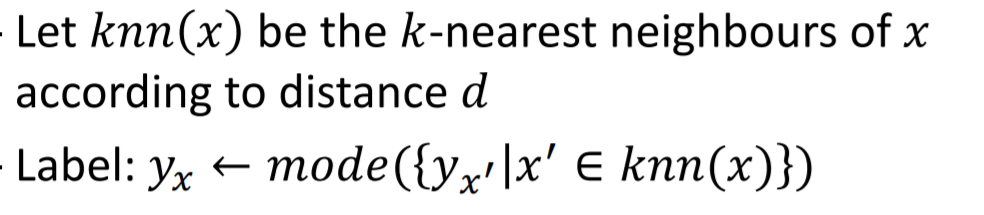Mathematical Perspective for the definition of KNN

In the above equation, we can see that the predicted y label is the mode of its nearby neighbors. For example, a similar analogy is applied to K-NN, we have a set of numbers like {5,5,5,3,3,2} then the mode of the set is 5, so the new data point will be equal to 5.

To perform K-NN, we think of data as vectors in high dimensional space. By applying geometric concepts like Euclidean Distance to Machine Learning Algorithms we can predict the labels of the data.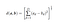Euclidean Distance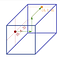Data points as vectors in high dimensional space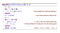Algorithm for K-NN

The above is the simple algorithm for K-NN this is how it is interpreted. We take an empty array S, compute all the distances from the test data point to all the data points. Store the distances in array S. Sort the values from the lowest distance to the highest distance. We select the k value based on the minimum distance from the test data point to the kth closest data point. Later, we obtain the label of the kth closest point to the test data point.

The question is how do we select the k, such that it is closest to the test data point. K values control the degree of smoothing to label the data for a classification problem. When we select K =1, it considers every data point nearby and labels that result in overfitting. That means the data point considers all the nearby labels and picks the labels at random. It may not be the correct prediction for that data point. Basically, this leads to high variance and low bais

When we select a larger K value, then the predictions will not be correct since it considers data points farther away from its vicinity. This leads to underfitting which can be said that it has high bias and low variance.

K value should be selected such that, it gives good accuracy on the unseen data. The below picture depicts the labeling of colors for different k values. We can observe K = 1 results in overfitting i.e., the degree of smoothing is sharp for all data points. When K = 3, it gives an optimum degree of smoothing where the data points are classified with better labeling. When K = 31 the degree of smoothing is very high which results in misclassification of data points.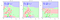Selection of K values

So, we need to select the K value such that, we get better predictions of the label on the data points, which gives better accuracy on the new data set. K value is chosen based on the training data set and not on the testing data set.

So, to solve this issue, we split the small portion training dataset as a validation dataset. So the split will be e.g., 60%, 20%, 20% respectively. On this dataset, we perform the K-NN model on training data and select the K value which gives the best accuracy on the validation dataset. The K value is selected then applied to the whole training data, then this K value is applied to test data to label the data.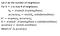Training, Validation and Test data split

Once we split the data into training, validation, and test data. The K value is selected and will remain constant in that case, the model will be tuned to some kind of predictions which leads to misclassification of data. To resolve this issue we use the cross-validation concept.

In cross-validation, we select K` value where we split the training data into K` subsets. We should assign one of the subsets as a validation dataset, and the others as training data, to get the K values. Later we juggle and shuffle each subset as a validation dataset, get a set of best values of K, out of which we select one K value to train and test the whole data.

By performing this method, we are increasing the size of the validation data and also increasing the robustness of the model. Basically, we covering all possible combinations to obtain the best K value. which is the hyperparameter and deciding factor to get the best working model.

K-NN algorithm is the most simple and to go algorithm in Machine learning. This algorithm has very good predictive power also, works best when it has relevant features in the dataset. However, It considers all features have the same importance, it cant pick the important ones in the data. Which makes it difficult to predict labels on various types of features. One more issue is the memory it takes to compute distances for each data point to all other data points.

To conclude, K-NN is one the friendliest algorithm in machine learning. It works with simple mathematical concepts that give robust predictions of labels. This works best when the features are relevant and small datasets.

Hi!! I graduated Master’s from the University of Waterloo, an MEng in Electrical and Computer Engineering. Happiness is learning a new thing every day.

## More from Sravani Subraveti

Hi!! I graduated Master’s from the University of Waterloo, an MEng in Electrical and Computer Engineering. Happiness is learning a new thing every day.